# RS Aggarwal Solutions for Class 9 Chapter 3: Factorisation of Polynomials Exercise 3F

## RS Aggarwal Solutions for Class 9 Maths Exercise 3F PDF

RS Aggarwal Solutions for Class 9 Maths Chapter 3 is one of the comprehensive study materials which can be used by students for a high academic score. Final exam preparations can be done faster in less time with better hold on concepts by using the solutions prepared by Mathematics experts. The answers are formulated in an elaborate manner with steps based on the CBSE syllabus evaluation method. The problems in RS Aggarwal textbook are based on the few repeated questions that have appeared in the previous year exams. RS Aggarwal Solutions for Class 9 Maths Chapter 3 Factorisation of Polynomials Exercise 3F are provided here.

## RS Aggarwal Solutions for Class 9 Chapter 3: Factorisation of Polynomials Exercise 3F Download PDF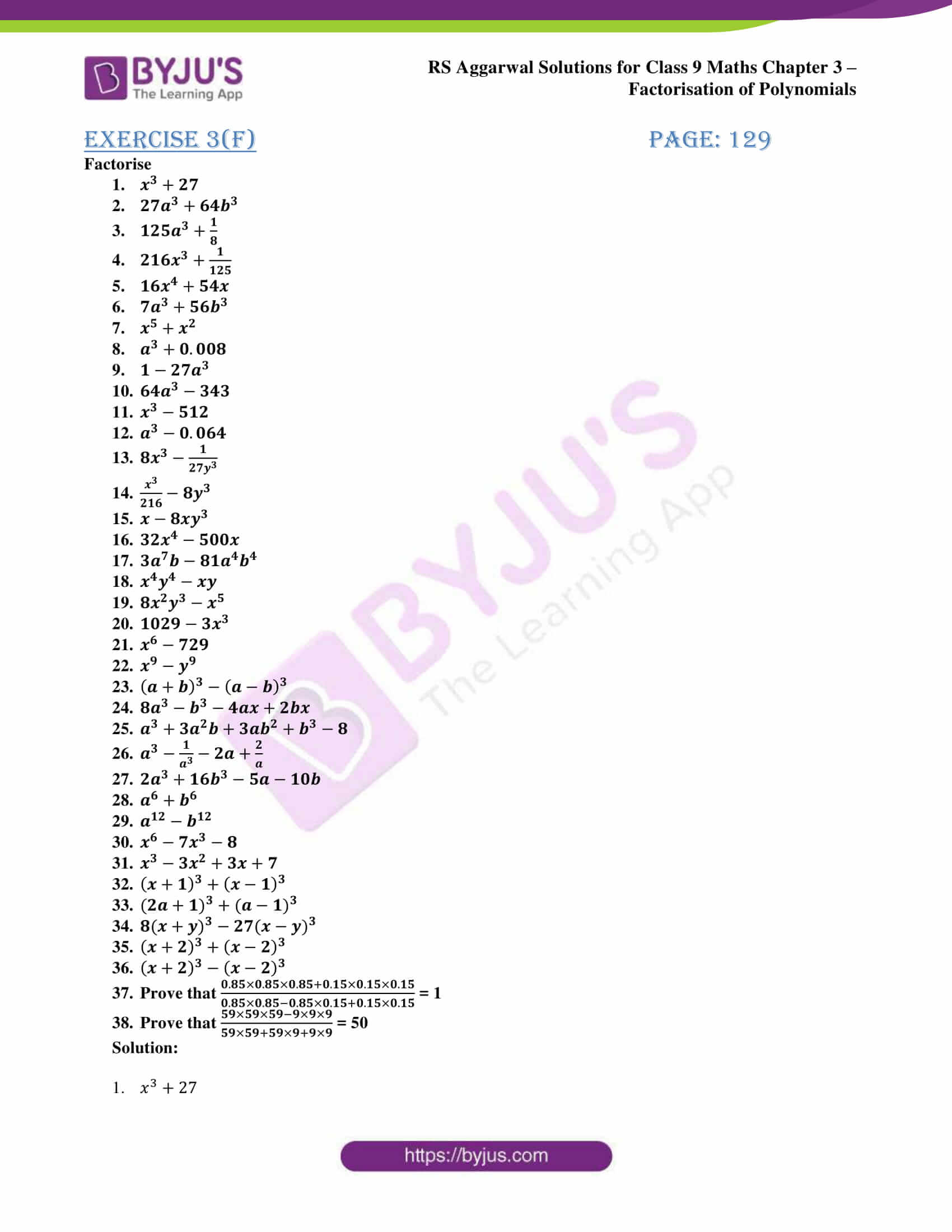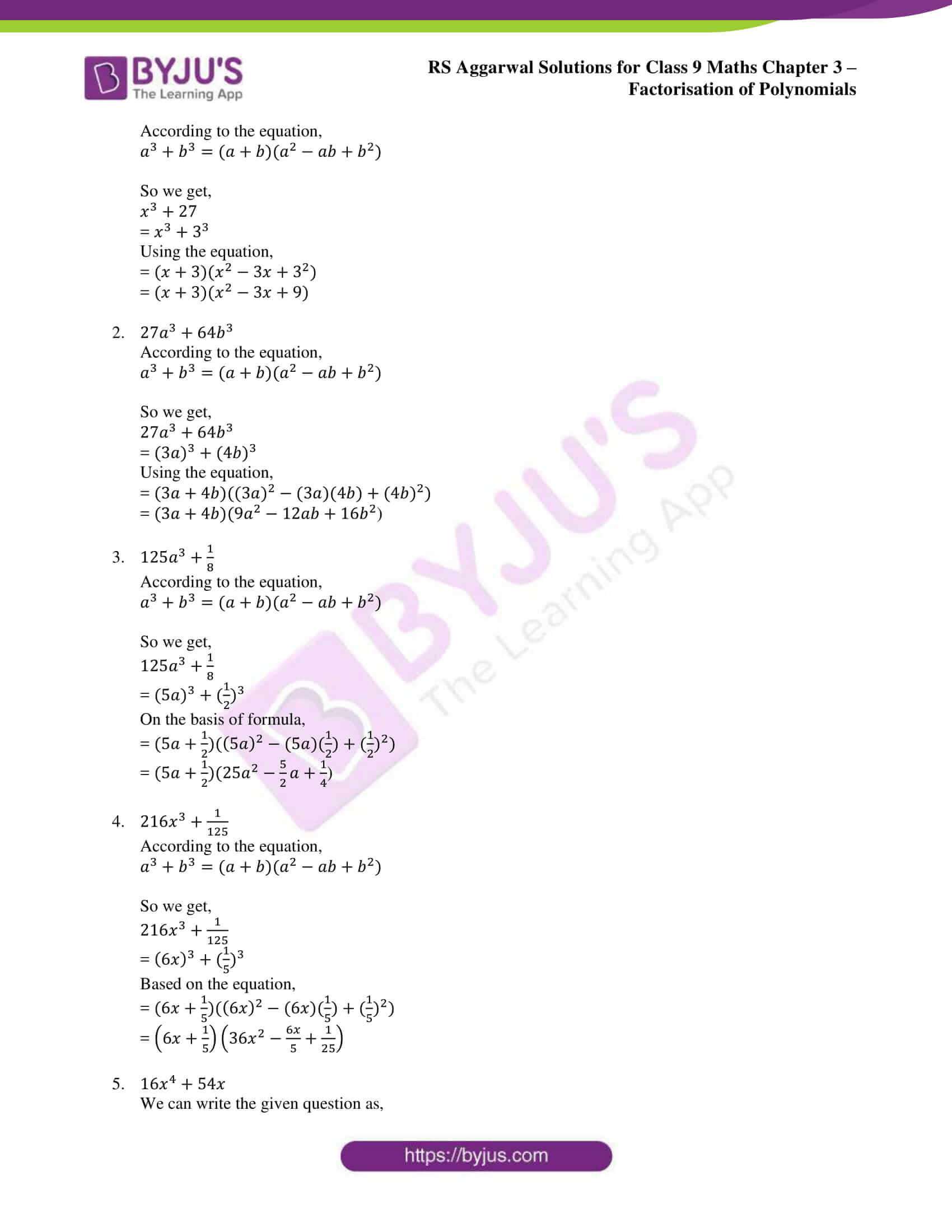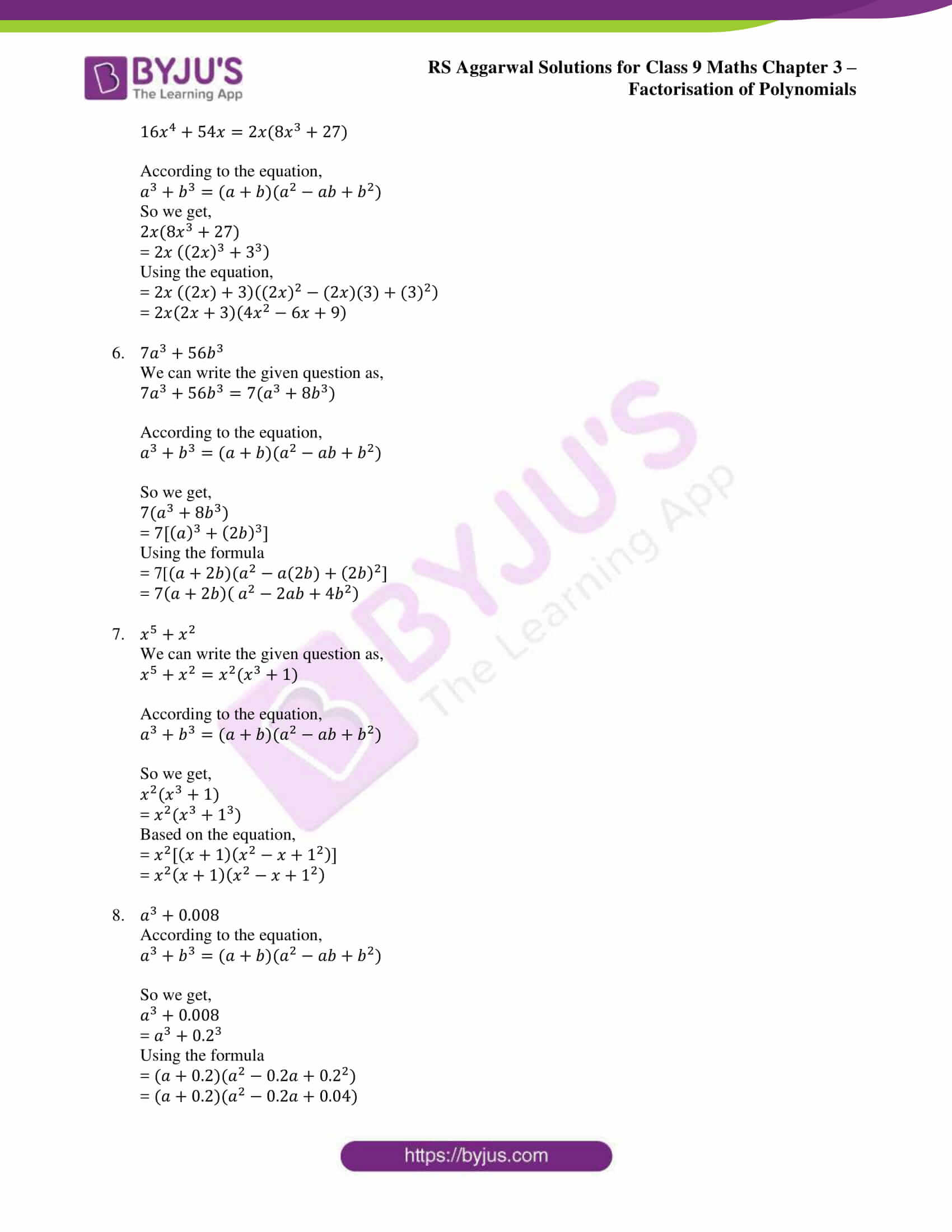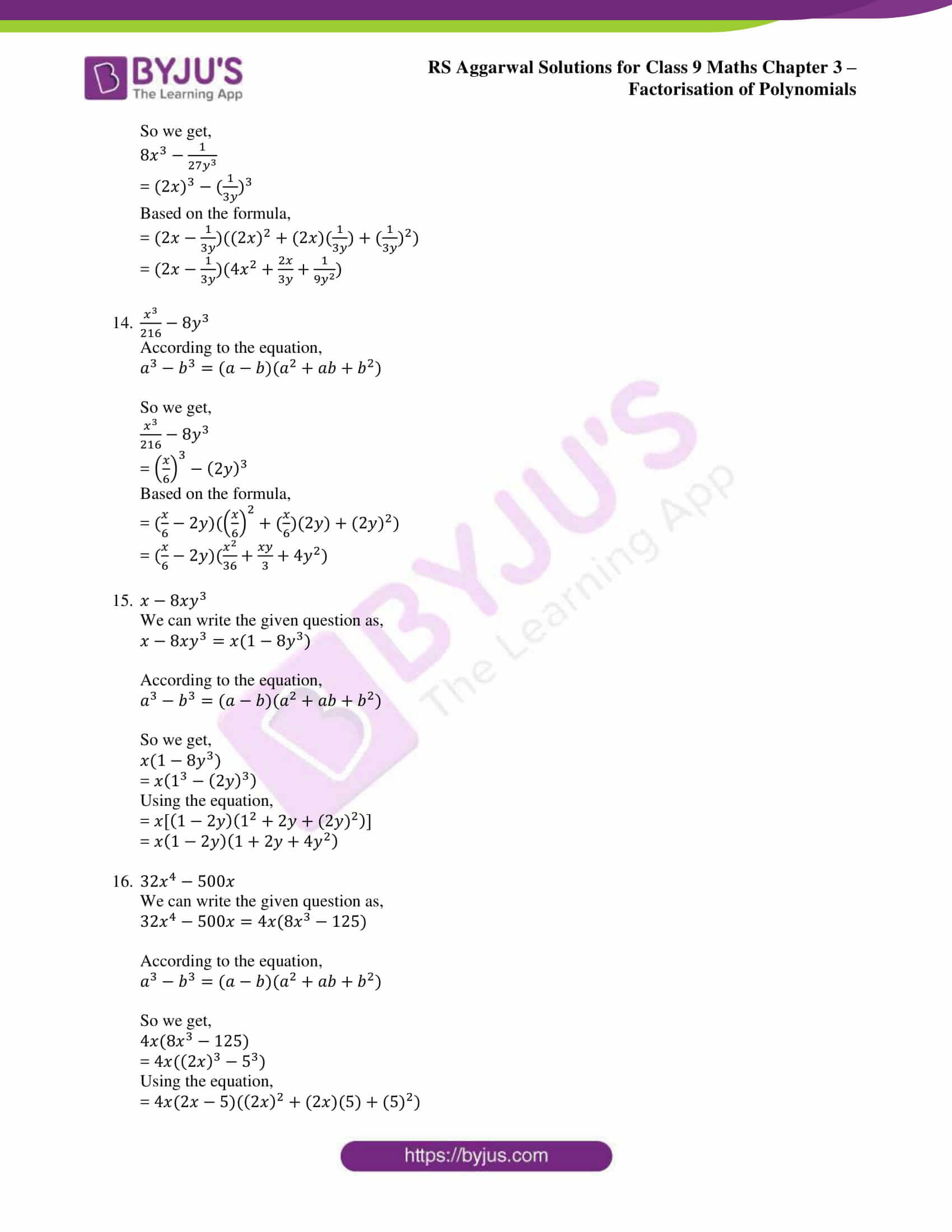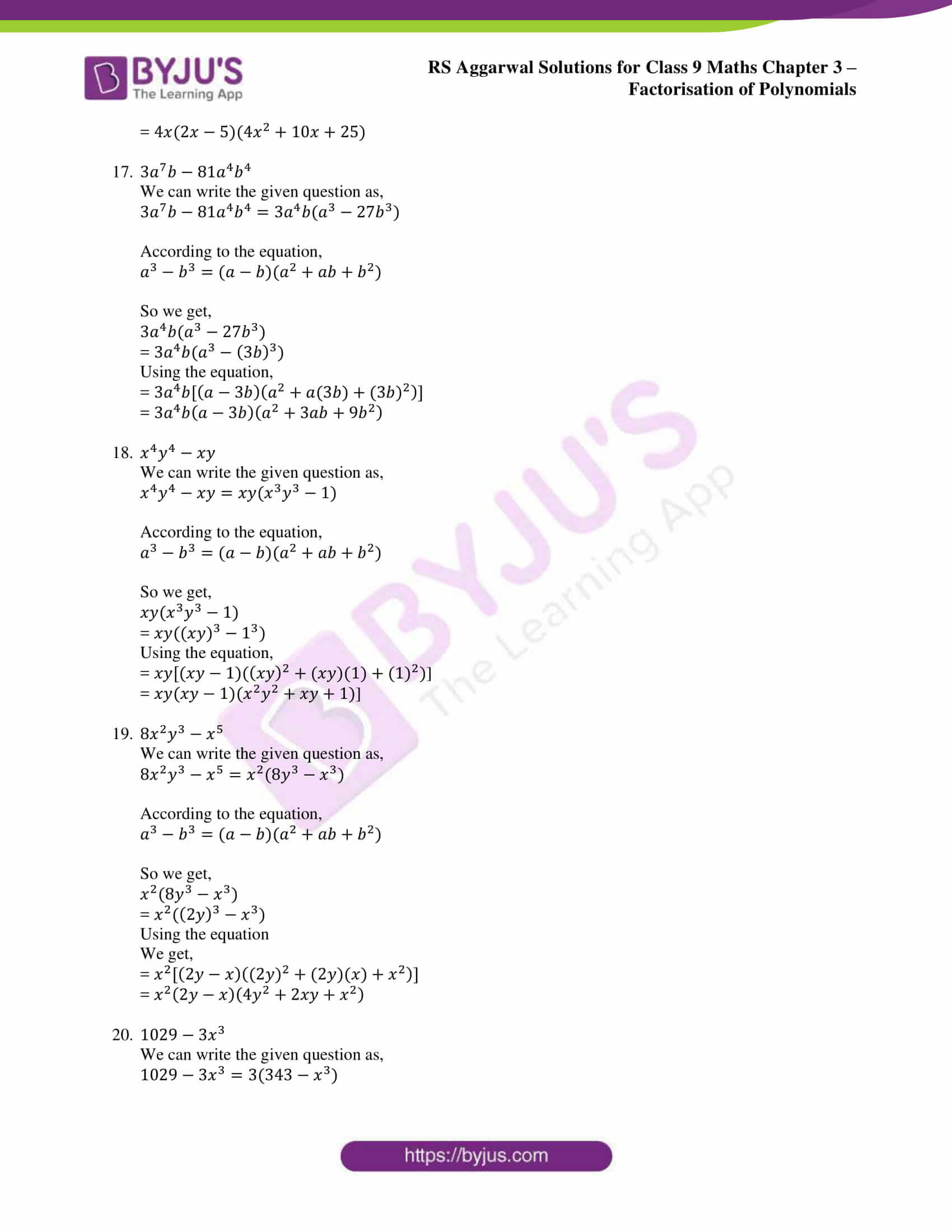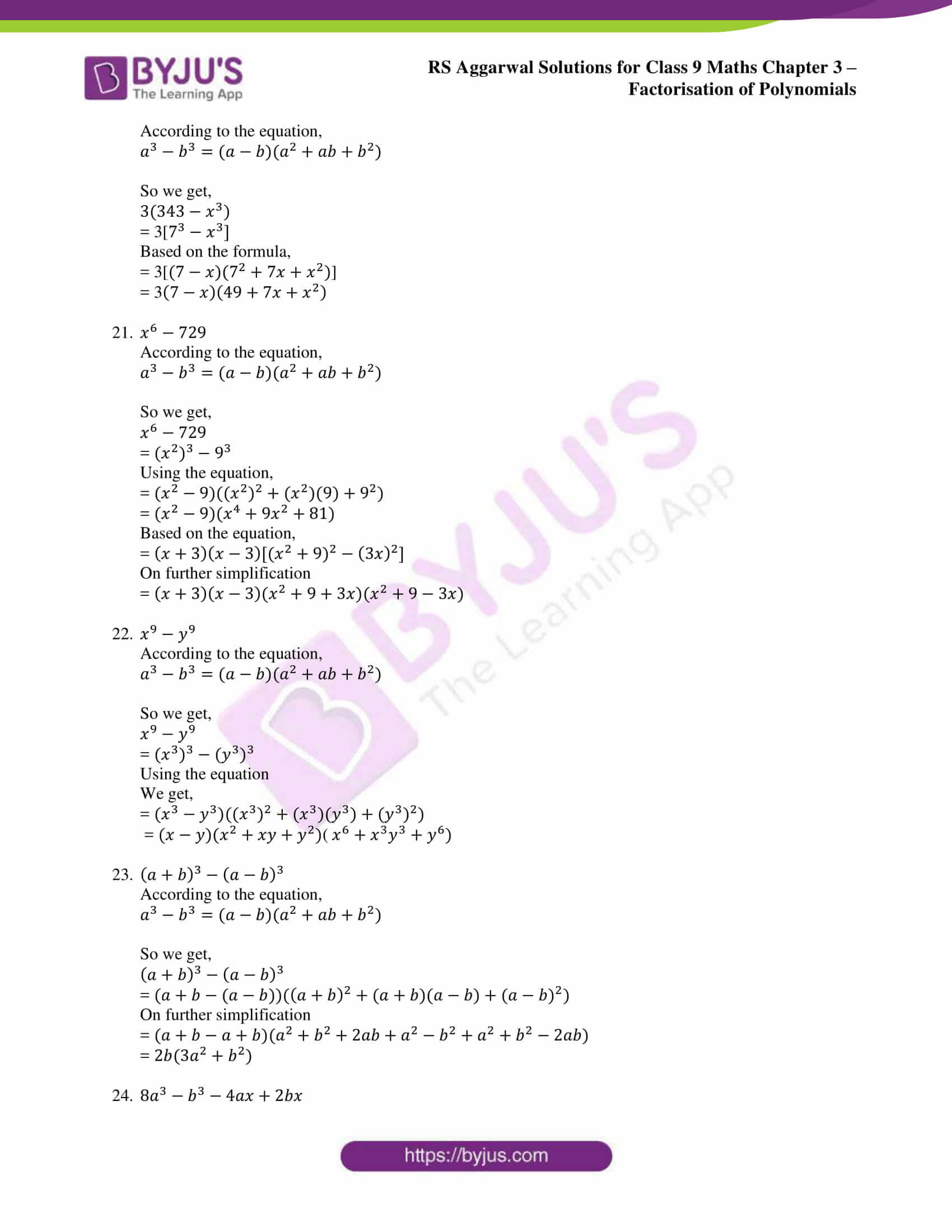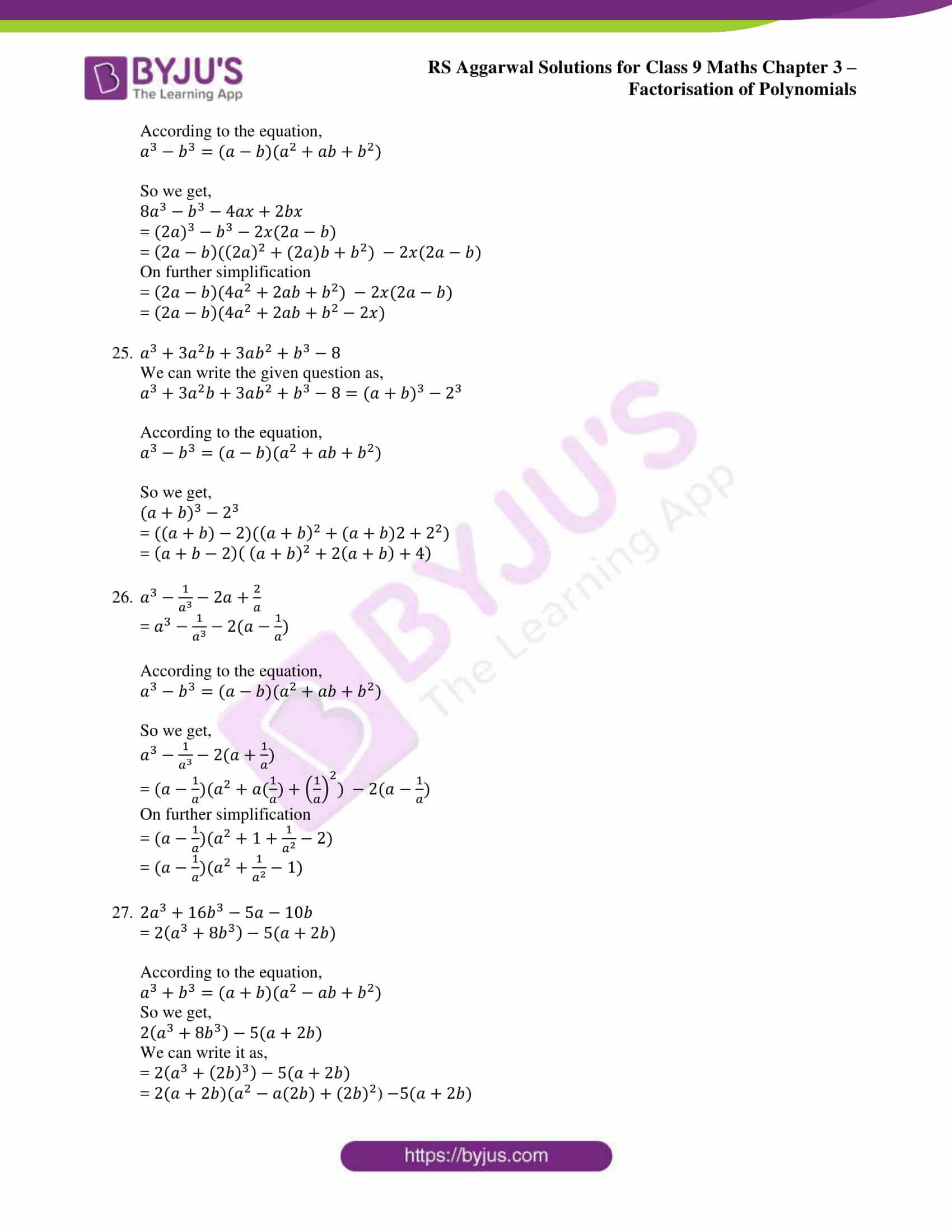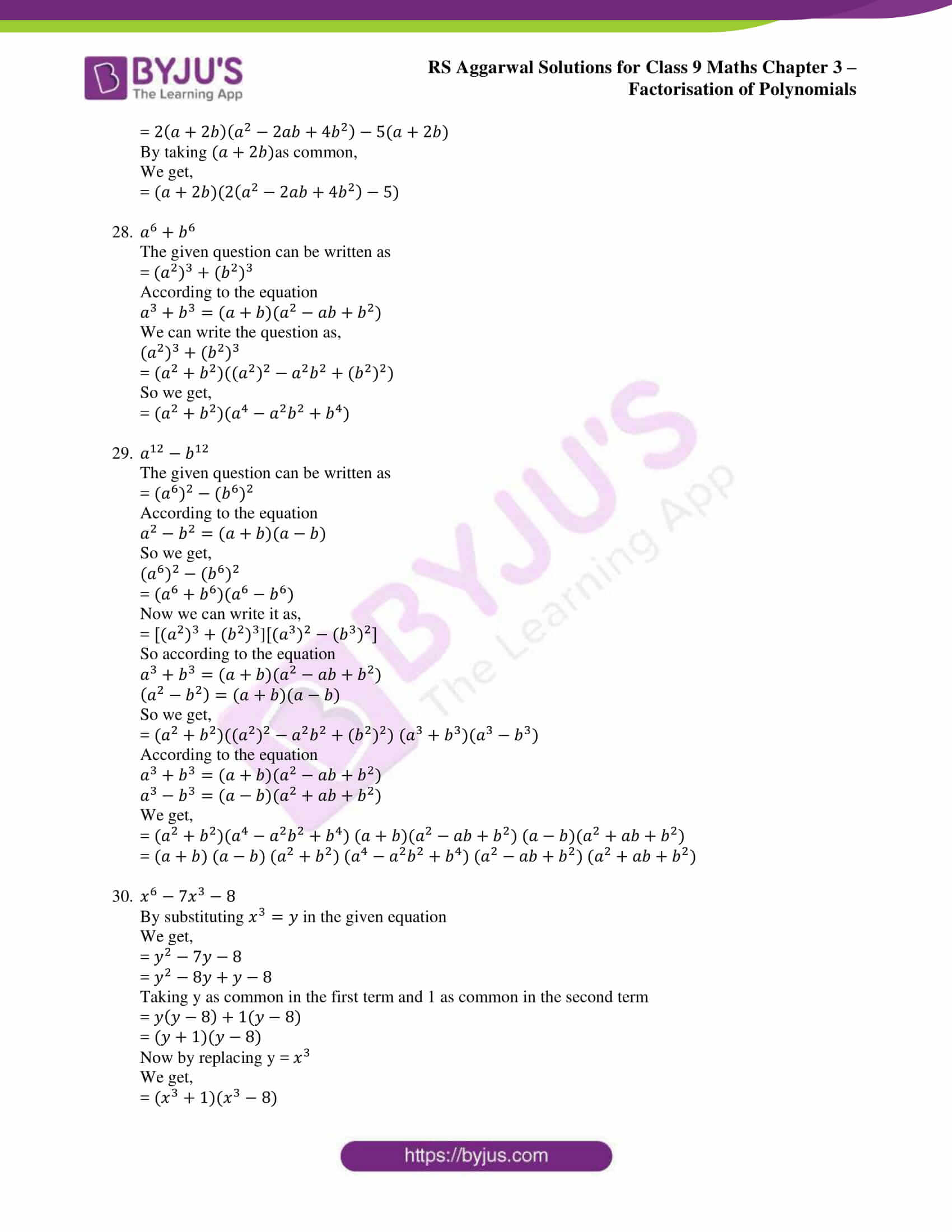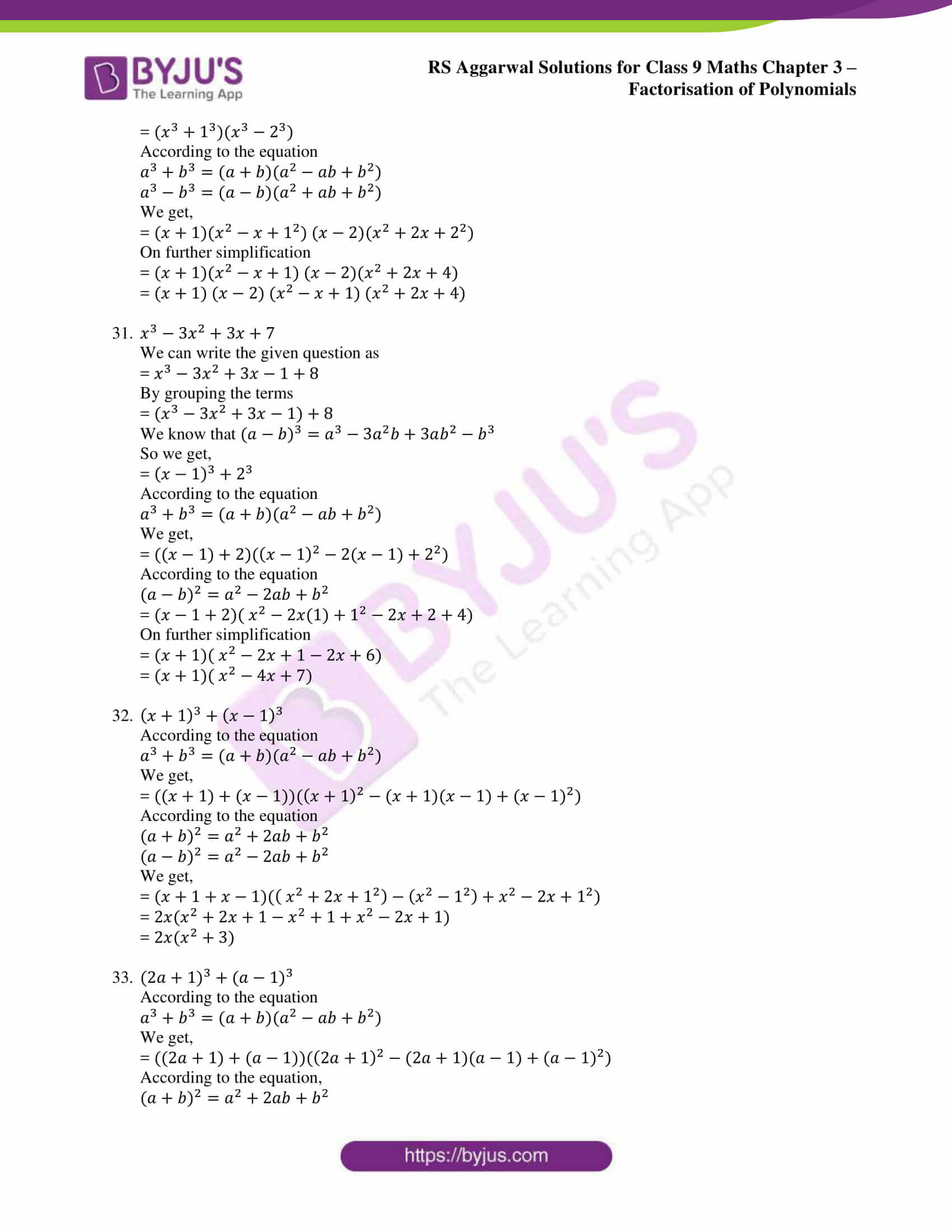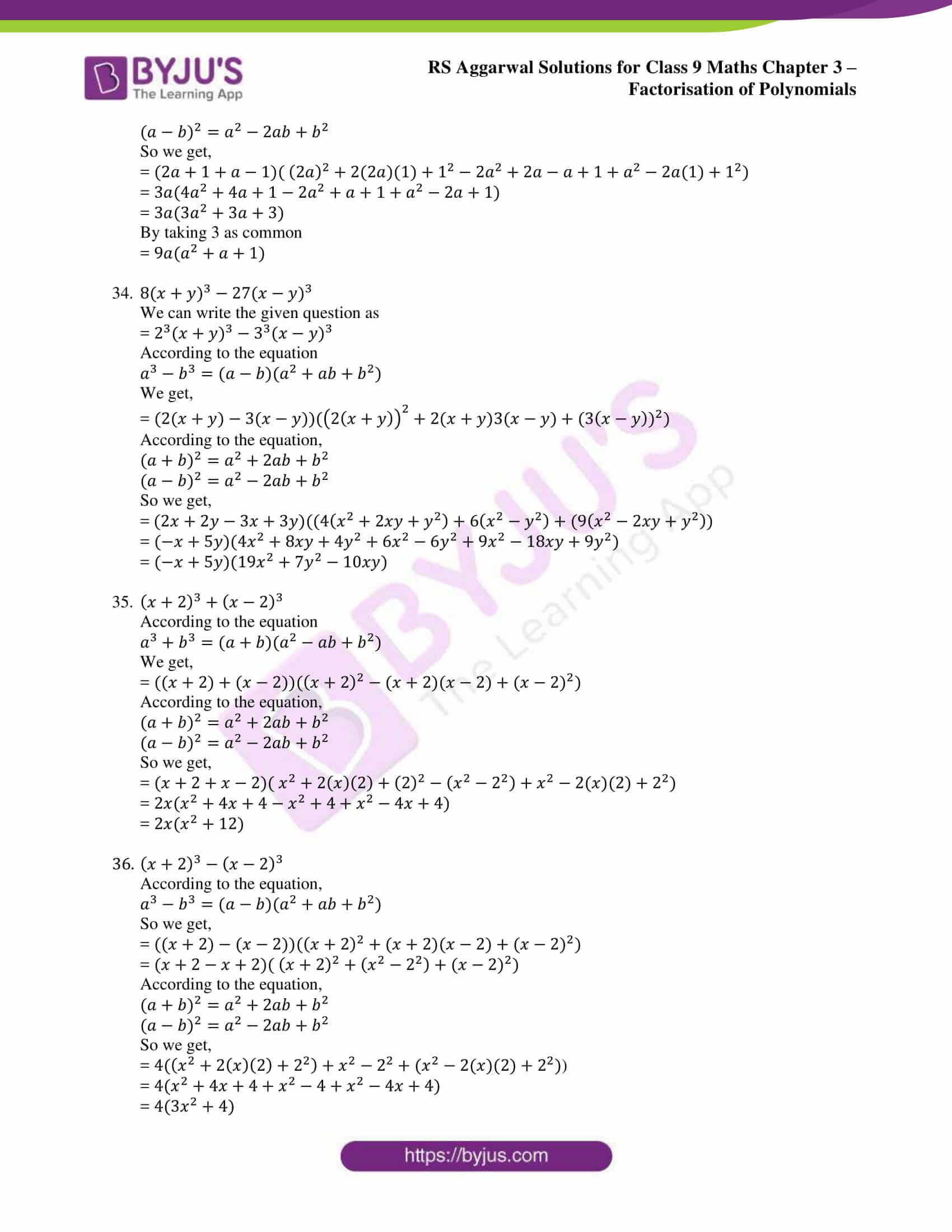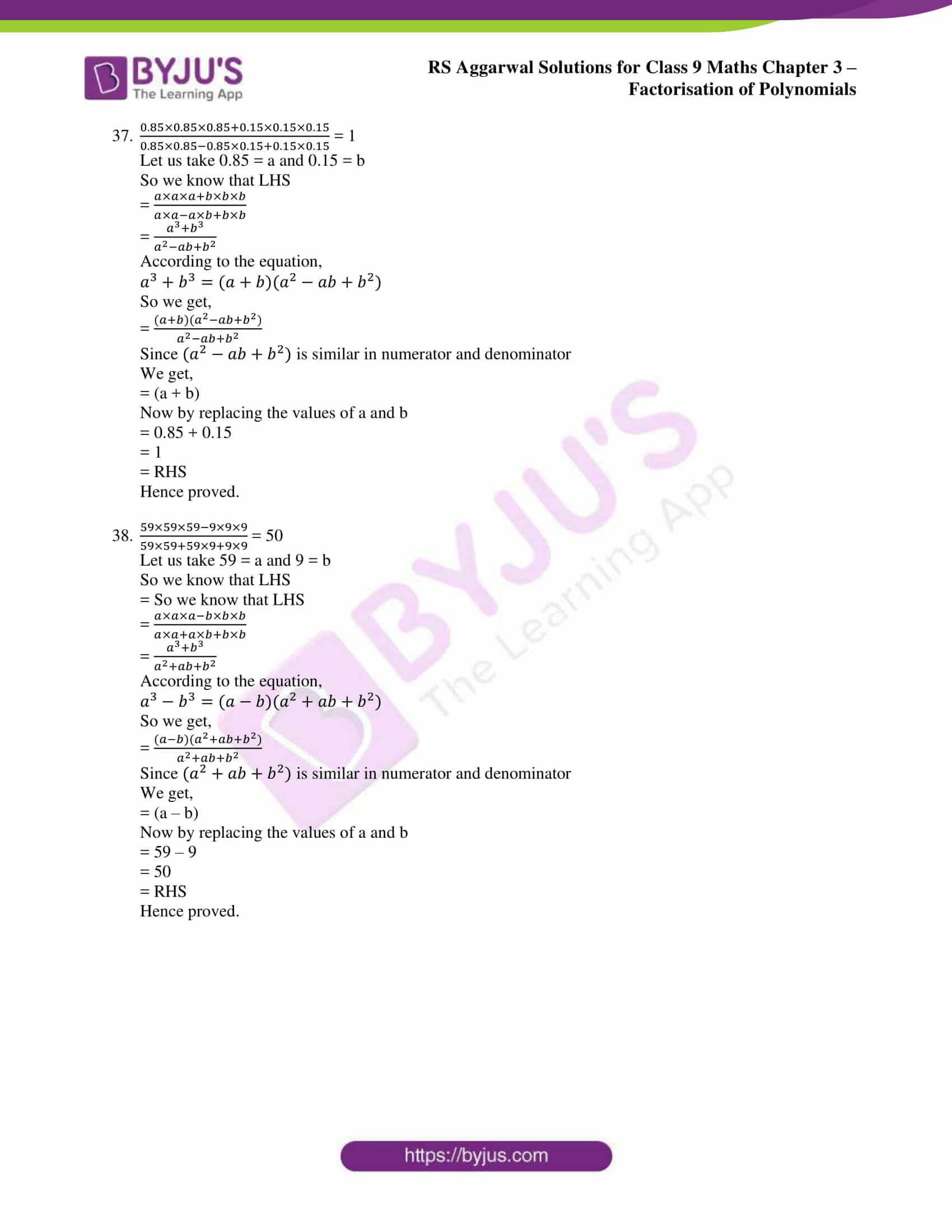### Access other exercise solutions of class 9 Maths Chapter 3: Factorisation of Polynomials

Exercise 3A Solutions 34 Questions

Exercise 3B Solutions 40 Questions

Exercise 3C Solutions 66 Questions

Exercise 3D Solutions 7 Questions

Exercise 3E Solutions 10 Questions

Exercise 3G Solutions 25 Questions

### RS Aggarwal Solutions Class 9 Maths Chapter 3 – Factorisation of Polynomials Exercise 3F

RS Aggarwal Solutions Class 9 Maths Chapter 3 Factorisation of Polynomials Exercise 3F has solved answers in PDF format, which are based on the Factorisation of Sum or Difference of Cubes.

### Key features of RS Aggarwal Solutions for Class 9 Maths Chapter 3: Factorisation of Polynomials Exercise 3F

• Doubts that arise in students while solving the problems can be cleared immediately.
• RS Aggarwal textbook is the main source for scoring good marks in the CBSE exams for Class 9.
• By downloading the PDF available on the BYJU’S website students can have an easy access whenever they are solving the chapters.
• To help students have a better grip on the concepts and master Mathematics is the major goal.